# Bohr’s Atomic Model

## Atomic Structure of Class 11

Bohr developed a model for hydrogen atom and hydrogen like one–electron species (hydrogenic species). He applied quantum theory in considering the energy of an electron bound to the nucleus.

Important postulates

• An atom consists of a dense nucleus situated at the centre with the electron revolving around it in circular orbits without emitting any energy. The force of attraction between the nucleus and an electron is equal to the centrifugal force of the moving electron.

• Of the finite number of circular orbits around the nucleus, an electron can revolve only in those orbits whose angular momentum (mvr) is an integral multiple of factor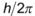mvr =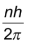where,m = mass of the electron

v = velocity of the electron

n = orbit number in which electron is present

r = radius of the orbit

• As long as an electron is revolving in an orbit it neither loses nor gains energy. Hence these orbits are called stationary states. Each stationary state is associated with a definite amount of energy and it is also known as energy levels. The greater the distance of the energy level from the nucleus, the more is the energy associated with it. The different energy levels are numbered as 1,2,3,4, ( from nucleus onwards) or K,L,M,N etc.

• Ordinarily an electron continues to move in a particular stationary state without losing energy. Such a stable state of the atom is called as ground state or normal state.

• If energy is supplied to an electron, it may jump (excite) instantaneously from lower energy (say 1) to higher energy level (say 2,3,4, etc.) by absorbing one or more quanta of energy. This new state of electron is called as excited state. The quantum of energy absorbed is equal to the difference in energies of the two concerned levels.

Since the excited state is less stable, atom will lose it’s energy and come back to the ground state.

Energy absorbed or released in an electron jump, (ΔE) is given by

ΔE = E2 – E1 = hν

where E2 and E1 are the energies of the electron in the first and second energy levels, and ν is the frequency of radiation absorbed or emitted.

[Note: If the energy supplied to hydrogen atom is less than 13.6 eV, it will accept or absorb only those quanta which can take it to a certain higher energy level i.e., all those photons having energy less than or more than a particular energy level will not be absorbed by hydrogen atom. But if energy supplied to hydrogen atom is more than 13.6 eV then all photons are absorbed and excess energy appear as kinetic energy of emitted photo electron].

### Radius and Energy levels of hydrogen atom

Consider an electron of mass ‘m’ and charge ‘e’ revolving around a nucleus of charge Ze (where, Z = atomic number and e is the charge of the proton) with a tangential
velocity v. r is the radius of the orbit in which electron is revolving.

By Coulomb’s Law, the electrostatic force of attraction between the moving electron
and nucleus is

Coulombic force =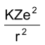K =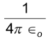(where ∈o is permittivity of free space)

K = 9 ×109 Nm2 C–2

In C.G.S. units, value of K = 1 dyne cm2 (esu)–2

The centrifugal force acting on the electron is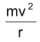Since the electrostatic force balance the centrifugal force, for the stable electron orbit.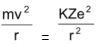… (1)

(or) v2 =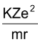… (2)

According to Bohr’s postulate of angular momentum quantization, we have

mvr =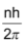v =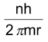v2 =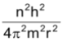… (3)

Equating (2) and (3)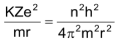solving for r we get r =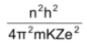where n = 1,2,3 - - - - - ∞

Hence only certain orbits whose radii are given by the above equation are available for the electron. The greater the value of n, i.e., farther the energy level from the nucleus the greater is the radius.

The radius of the smallest orbit (n=1) for hydrogen atom (Z=1) is ro.

ro =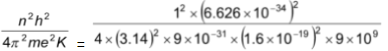= 5.29 ×10–11 m = 0.529 Å

Radius of nth orbit for an atom with atomic number Z is simply written as

rn = 0.529 ×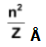Calculation of energy of an electron

The total energy, E of the electron is the sum of kinetic energy and potential energy.

Kinetic energy of the electron = ½ mv2

Potential energy =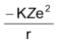Total energy = 1/2 mv2 –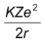… (4)

From equation (1) we know that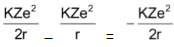∴ ½ mv2 =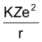Substituting this in equation (4)

Total energy (E) =Substituting for r, gives us

E =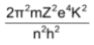where n = 1,2,3……….

This expression shows that only certain energies are allowed to the electron. Since this energy expression consist of so many fundamental constant, we are giving you the following simplified expressions.

E = –21.8 ×10–12 ×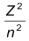erg per atom

= –21.8 ×10–19 ×J per atom = –13.6 ×eV per atom

(1eV = 3.83 ×10–23 kcal

1eV = 1.602 ×10–12 erg

1eV = 1.602 ×10–19J)

E = –313.6 ×kcal / mole (1 cal = 4.18 J)

The energies are negative since the energy of the electron in the atom is less than the energy of a free electron (i.e., the electron is at infinite distance from the nucleus) which is taken as zero. The lowest energy level of the atom corresponds to n=1, and as the quantum number increases, E becomes less negative.

When n = ∞, E = 0, which corresponds to an ionized atom i.e., the electron and nucleus are infinitely separated.

H → H++ e– (ionisation).

### Explanation for hydrogen spectrum by Bohr’s theory

According to the Bohr’s theory electron neither emits nor absorbs energy as long as it stays in a particular orbit. However, when an atom is subjected to electric discharge or high temperature, and electron in the atom may jump from the normal energy level, i.e., ground state to some higher energy level i.e, exited state. Since the life time of the electron in excited state is short, it returns to the ground state in one or more jumps.

During each jump, energy is emitted in the form of a photon of light of definite wavelength or frequency. The frequency of the photon of light thus emitted depends upon the energy difference of the two energy levels concerned (n1, n2) and is given by

hν = E2 – E1 =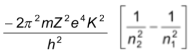ν =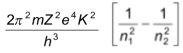The frequencies of the spectral lines calculated with the help of above equation are found to be in good agreement with the experimental values. Thus, Bohr’s theory elegantly explains the line spectrum of hydrogen and hydrogenic species.

## Bohr had calculated Rydberg constant from the above equation.

ν =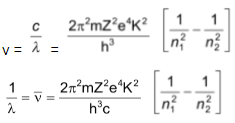where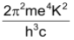= 1.097 × 10–7m–1 or 109678 cm–1

i.e., Rydberg constant (R)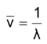= RZ2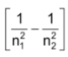According to quantum mechanics the Rydberg constant is given by R =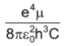Were μ is reduced mass for the atom ε0 is the permitivity of a vacuum. The reduced mass of an atom with one electron is given by

μ =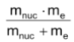where mnuc = mass of nucleus

and me = mass of electron. For a nucleus with mass mnuc → α

μ = me

and Rα = 109673 cm–1

Calculation of velocity

We know that

mvr =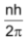; v =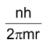By substituting for r we are getting

v =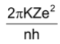Where excepting n and z all are constants

v = 2.18 ×108  Z/n  cm/sec.

Further application of Bohr’s work was made, to other one electron species (Hydrogenic ion) such as He+ and Li2+. In each case of this kind, Bohr’s prediction of the spectrum was correct.

## Merits of Bohr’s theory

i) The experimental value of radii and energies in hydrogen atom are in good agreement with that calculated on the basis of Bohr’s theory.

ii) Bohr’s concept of stationary state of electron explains the emission and absorption spectra of hydrogen like atoms.

iii) The experimental values of the spectral lines of the hydrogen spectrum are in close agreement with that calculated by Bohr’s theory.

### Limitations of Bohr’s theory

i) It does not explain the spectra of atoms having more than one electron.

ii) Bohr’s atomic model failed to account for the effect of magnetic field (Zeeman effect) or electric field (Stark effect) on the spectra of atoms or ions. It was observed that when the source of a spectrum is placed in a strong magnetic or electric field, each spectral line further splits into a number of lines. This observation could not be explained on the basis of Bohr’s model.

iii) De Broglie suggested that electrons like light have dual character. It has particle and wave character. Bohr treated the electron only as particle.

iv) Another objection to Bohr’s theory came from Heisenberg’s Uncertainty Principle. According to this principle “It is impossible to determine simultaneously the exact position and momentum of a small moving particle like an electron”. The postulate of Bohr, that electrons revolve in well defined orbits around the nucleus with well defined velocities is thus not tenable.

### Quantum Numbers

An atom contains large number of shells and subshells. These are distinguished from one another on the basis of their size, shape and orientation (direction) in space. The parameters are expressed in terms of different numbers called quantum numbers.

Quantum numbers may be defined as a set of four numbers with the help of which we can get complete information about all the electrons in an atom. It tells us the address of the electron i.e., location, energy, the type of orbital occupied and orientation
of that orbital.

i) Principal quantum number (n): It tells the main shell in which the electron resides and the approximate distance of the electron from the nucleus. It also tells the maximum number of electrons a shell can accommodate is 2n2, where n is the principal quantum number.

### Shell KLMN

Principal quantum number (n) 1234

Maximum number of electrons 281832

ii) Azimuthal or angular momentum quantum number (l): This represents the number of subshells present in the main shell. These subsidiary orbits within a shell will be denoted as 1,2,3,4,… or s,p,d,f… This tells the shape of the subshells. The orbital angular momentum of the electron is given as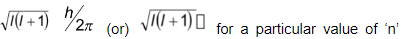(or)  for a particular value of ‘n’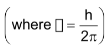. For a given value of n values of possible l vary from 0 to n – 1.

iii) The magnetic quantum number (m):An electron due to its angular motion around the nucleus generates an electric field. This electric field is expected to produce a magnetic field. Under the influence of external magnetic field, the electrons of a subshell can orient themselves in certain preferred regions of space around the nucleus called orbitals. The magnetic quantum number determines the number of preferred orientations of the electron present in a subshell. The values allowed depends on the value of l, the angular momentum quantum number, m can assume all integral values between –l to +l including zero. Thus m can be –1, 0, +1 for l = 1. Total values of m associated with a particular value of l is given by 2l + 1.

iv) The spin quantum number (s): Just like earth not only revolves around the sun but also spins about its own axis, an electron in an atom not only revolves around the nucleus but also spins about its own axis. Since an electron can spin either in clockwise direction or in anticlockwise direction, therefore, for any particular value of magnetic quantum number, spin quantum number can have two values, i.e., +1/2 and –1/2 or these are represented by two arrows pointing in the opposite directions, i.e., ↑ and ↓. When an electron goes to a vacant orbital, it can have a clockwise or anti clockwise spin i.e., +1/2 or –1/2. This quantum number helps to explain the magnetic properties of the substances.

### Shapes and size of orbitals

An orbital is the region of space around the nucleus within which the probability of finding an electron of given energy is maximum (90–95%). The shape of this region (electron cloud) gives the shape of the orbital. It is basically determined by the azimuthal quantum number l, while the orientation of orbital depends on the magnetic quantum number (m). Let us now see the shapes of orbitals in the various subshells.

 s–orbitals: These orbitals are spherical and symmetrical about the nucleus. The probability of finding the electron is maximum near the nucleus and keep on decreasing as the distance from the nucleus increases. There is vacant space between two successive s–orbitals known as radial node. But there is no radial node for 1s orbital since it is starting from the nucleus.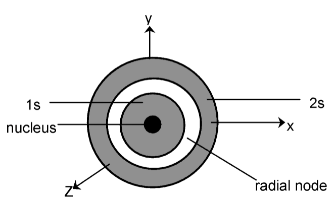The size of the orbital depends upon the value of principal quantum number(n). Greater the value of n, larger is the size of the orbital. Therefore, 2s–orbital is larger than 1s orbital but both of them are non-directional and spherically symmetrical in shape.

p–orbitals (l =1): The probability of finding the p–electron is maximum in two lobes on the opposite sides of the nucleus. This gives rise to a dumb–bell shape for the p–orbital. For p–orbital l = 1. Hence, m = –1, 0, +1. Thus, p–orbital have three different orientations. These are designated as px,py & pz depending upon whether the density of electron is maximum along the x y and z axis respectively. As they are not spherically symmetrical, they have directional character. The two lobes of p–orbitals are separated by a nodal plane, where the probability of finding electron is zero.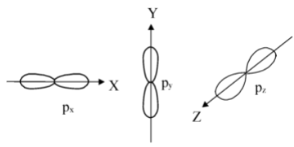The three p-orbitals belonging to a particular energy shell have equal energies and are called degenerate orbitals.

d–orbitals (l =2): For d–orbitals, l =2. Hence m=–2,–1,0,+1,+2. Thus there are 5 d orbitals. They have relatively complex geometry. Out of the five orbitals, the three (dxy, dyz,dzx) project in between the axis and the other two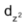and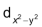lie along the axis.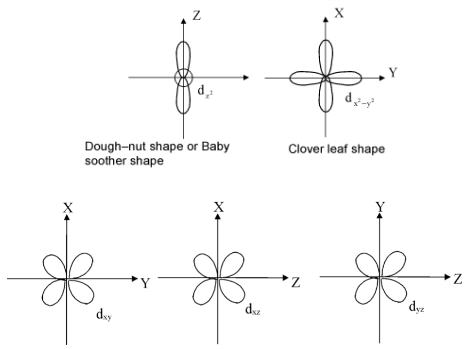### Rules for filling of electrons in various orbitals

The atom is built up by filling electrons in various orbitals according to the following rules.

Aufbau Principle: This principle states that the electrons are added one by one to the various orbitals in order of their increasing energy starting with the orbital of lowest energy. The increasing order of energy of various orbital is

1s,2s,2p,3s,3p,4s,3d,4p,5s,4d,5p,6s,4f,5d,6p,5f,6d,7p……………………

How to remember such a big sequence? To make it simple we are giving you the method to write the increasing order of the orbitals. Starting from the top, the direction of the arrows gives the order of filling of orbitals.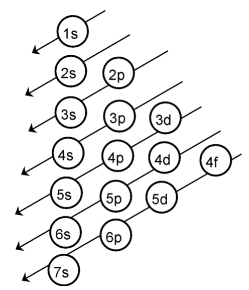Alternatively, the order of increasing energies of the various orbitals can be calculated on the basis of (n+l) rule.

The energy of an orbital depends upon the sum of values of the principal quantum number (n) and the azimuthal quantum number (l). This is called (n+ l) rule.
According to this rule,

“In neutral isolated atom, the lower the value of (n+ l) for an orbital, lower is its energy. However, if the two different types of orbitals have the same value of
(n+ l), the orbitals with lower value of n has lower energy’’.

Illustration of (n + l) rule

 Type of orbitals Value of n Values of l Values of (n+ l) Relative energy 1s 1 0 1+0=1 Lowest energy 2s 2 0 2+0=2 Higher energy than 1s orbital 2p 2 1 2+1=3 2p orbital (n=2) have lower energy than 3s orbital (n=3) 3s 3 0 3+1=3

### Pauli’s Exclusion principle

According to this principle, an orbital can contain a maximum number of two electrons and these two electrons must be of opposite spin.

Two electrons in an orbital can be represented by

↑↓ or ↓↑

## Hund’s rule of maximum multiplicity

This rule deals with the filling of electrons in the equal energy (degenerate) orbitals of the same sub shell (p,d and f). According to this rule,

“Electron pairing in p,d and f orbitals cannot occur untill each orbital of a given subshell contains one electron each or is singly occupied”.

This is due to the fact that electrons being identical in charge, repel each other when present in the same orbital. This repulsion can, however, be minimised if two electrons move as far apart as possible by occupying different degenerate orbitals. All the electrons in a degenerate set of orbitals will have same spin.

### Electronic configuration of elements

Electronic configuration is the distribution of electrons into different shells, subshells and orbitals of an atom .

Keeping in view the above mentioned rules, electronic configuration of any orbital can be simply represented by the notation.]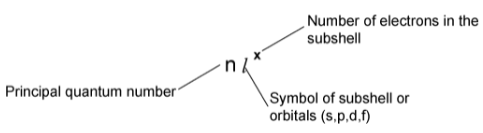Alternatively

Orbital can be represented by a box and an electron with its direction of spin by arrow. To write the electronic configuration, just we need to know (i) the atomic number (ii) the order in which orbitals are to be filled (iii) maximum number of electrons in a shell, sub–shell or orbital.

a) Each orbital can accommodate two electrons

b) The number of electrons to be accomodated in a subshell is 2 × number of
degenerate orbitals.

 Subshell Maximum number of electrons s 2 p 6 d 10 f 14

c) The maximum number of electron in each shell (K,L,M,N…) is given by 2n2.
where n is the principal quantum number.

d) The maximum number of orbitals in a shell is given by n2 where n is the principal quantum number.

e) The number of nodal planes associated with an orbital is given by l -1.

Importance of knowing the electronic configuration

The chemical properties of an element are dependent on the relative arrangement
of its electrons.

### Exceptional Configurations

Stability of half filled and completely filled orbitals

Cu has 29 electrons. Its expected electronic configuration is

1s22s22p63s23p64s23d9

But a shift of one electron from lower energy 4s orbital to higher energy 3d orbital will make the distribution of electron symmetrical and hence will impart more stability.

Thus the electronic configuration of Cu is

1s2 2s22p63s23p64s13d10

Fully filled and half filled orbitals are more stable.